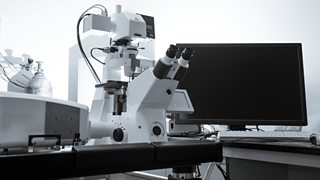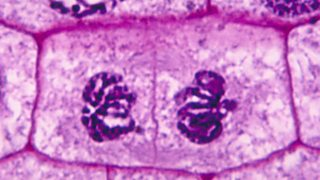# Under the microscopeA modern light microscope

Microscopes are used to study cells. Modern light microscopes can magnify images about 1500 times, while electron microscopes can magnify images about two million times.

## Calculating magnification

The of a biological specimen is calculated using this equation:

$magnification =\frac{measured~size}{actual~size}$

Question

A plant cell in a photograph measures 15 mm across. If the actual size of the cell is 0.015 mm, what is the magnification in the photograph?

$magnification = \frac{measured~size}{actual~size}=\frac{15~mm}{0.015~mm}=\times~1000$Plant cells seen through a microscope

## Calculating actual size

The actual size of a biological specimen is calculated using this equation:

$actual~size =\frac{measured~size}{magnification}$

Question

The nucleus in a photograph of a cell measures 3 mm across. If the magnification in the photograph is × 500, what is the actual size of the nucleus?

$actual~size = \frac{measured~size}{magnification}= \frac{3~mm}{500}= 0.006~mm$

Move on to Test